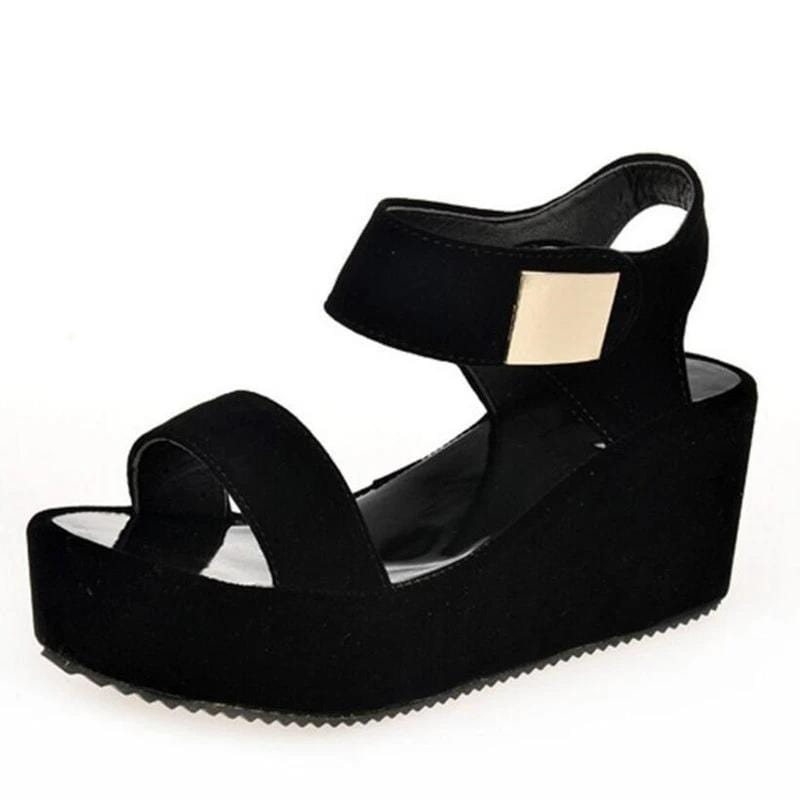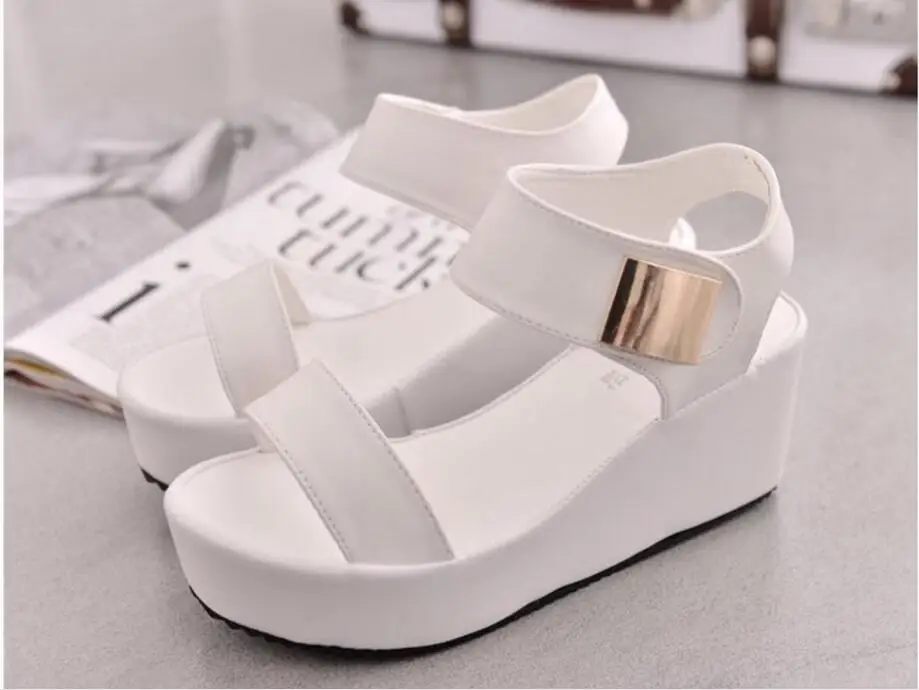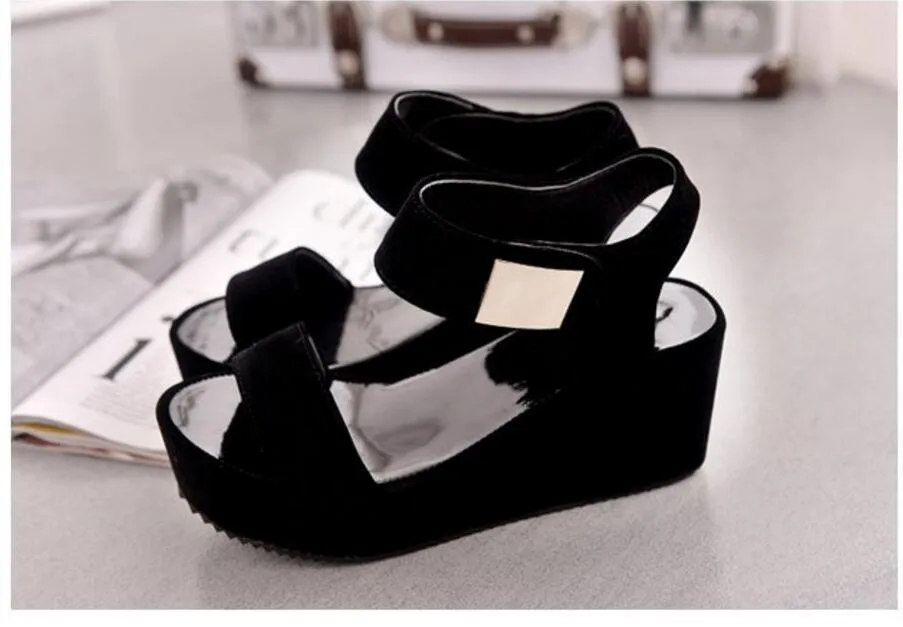# Women`s Roman Gladiator peep-toe flat sandals

Sale price \$24.99 Regular price \$44.9934 = 22cm = 4 United States

35 = 22.5cm = 5 United States

36 = 23cm = 6 United States

37 = 23.5cm = 6.5 United States

38 = 24cm = 7.5 United States

39 = 24.5cm = 8.5 United States

40 = 25cm = 9 United States

41 = 25.5cm = 9.5 United States

42 = 26cm = 10 United States

43 = 26.5cm = 10.5 United States

44 = 27cm = 11 United States

45 = 27.5cm = 11.5 United States

46 = 28cm = 12 United States

47 = 28.5cm = 12.5 U.S.

48 = 29cm = 13 United States